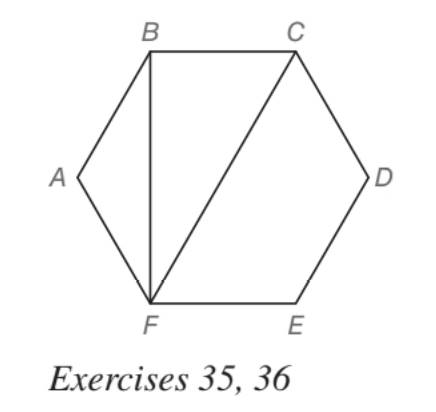Chapter 5.5, Problem 36EElementary Geometry For College St...

7th Edition
Alexander + 2 others
ISBN: 9781337614085

Solutions

Chapter
SectionElementary Geometry For College St...

7th Edition
Alexander + 2 others
ISBN: 9781337614085
Textbook Problem

In regular hexagon A B C D E F , the length of A B is x centimeters. In terms of x find the length of a) diagonal B F ¯ . b) diagonal C F ¯ .To determine

a) To find:

The exact length of the diagonal BF¯ of the regular hexagon ABCDEF and with AB=x cm.

Explanation

Approach:

For a right triangle, for which the measure of the interior angles 30°, 60°, and 90°; if ‘a’ is the length of measure of the shorter leg; opposite to the angle 30°, then the length of the other two sides is given by

Length of the longer leg (opposite to 60°) =a3

Length of the hypotenuse (opposite to 90°)=2a.

In general

Length of the longer leg =3× (Length of the shorter leg)

Length of the hypotenuse =2× (Length of the shorter leg)

Calculation:

Given,

A regular hexagon ABCDEF with the diagonal BF and of one of he face length measures AB=x cm.

Since, we have a regular hexagon, all the six faces are congruent and also all the six face angles,

AB=BC=CD=DE=EF=FA=x cm.

A=B=C=D=E=F=n-2·180°n

=6-2·180°6

=4·180°6

=4·30°

=120°

Thus, the measure of the length of each face of the polygon is x cm.

The measure of the each face angle of the polygon is 120°.

Here, the diagonal BF is perpendicular to the sides BC and EF of the hexagon.

Thus, the angles B and F of the ABF is given by

B=F=120°-90°

B=F=30°

Consider the ABF of the hexagon shown above and draw the auxiliary line AP¯BF¯, which will bisect both the angle A of the triangle and also the base BF of the as such ABF is an isosceles triangle with AB¯AF¯

To determine

b) To find:

The exact length of the diagonal CF¯ of the regular hexagon ABCDEF and with AB=x cm.

Still sussing out bartleby?

Check out a sample textbook solution.

See a sample solution

The Solution to Your Study Problems

Bartleby provides explanations to thousands of textbook problems written by our experts, many with advanced degrees!

Get Started

Solve the equations in Exercises 112 for x (mentally, if possible). x1=cx+d(c1)

Finite Mathematics and Applied Calculus (MindTap Course List)

The polar coordinates of the point P in the figure at the right are:

Study Guide for Stewart's Single Variable Calculus: Early Transcendentals, 8th

Find the unit tangent vector for at t = –1.

Study Guide for Stewart's Multivariable Calculus, 8th

In Exercises 1-8, determine which of the matrices are regular. [10.800.2]

Finite Mathematics for the Managerial, Life, and Social Sciences